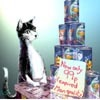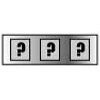# Resources tagged with: Triangle numbers

Filter by: Content type:
Age range:
Challenge level:

There are 21 NRICH Mathematical resources connected to Triangle numbers, you may find related items under Properties of Numbers.

Broad Topics > Properties of Numbers > Triangle numbers### Handshakes

##### Age 11 to 14 Challenge Level:

Can you find an efficient method to work out how many handshakes there would be if hundreds of people met?### Mystic Rose

##### Age 14 to 16 Challenge Level:

Use the animation to help you work out how many lines are needed to draw mystic roses of different sizes.### Triangle Numbers

##### Age 11 to 14 Challenge Level:

Take a look at the multiplication square. The first eleven triangle numbers have been identified. Can you see a pattern? Does the pattern continue?### Route to Infinity

##### Age 11 to 14 Challenge Level:

Can you describe this route to infinity? Where will the arrows take you next?### Factors and Multiples Puzzle

##### Age 11 to 14 Challenge Level:

Using your knowledge of the properties of numbers, can you fill all the squares on the board?### Take Ten Sticks

##### Age 11 to 16 Challenge Level:

Take ten sticks in heaps any way you like. Make a new heap using one from each of the heaps. By repeating that process could the arrangement 7 - 1 - 1 - 1 ever turn up, except by starting with it?### Picturing Triangular Numbers

##### Age 11 to 14 Challenge Level:

Triangular numbers can be represented by a triangular array of squares. What do you notice about the sum of identical triangle numbers?### Iff

##### Age 14 to 18 Challenge Level:### Summing Consecutive Numbers

##### Age 11 to 14 Challenge Level:

15 = 7 + 8 and 10 = 1 + 2 + 3 + 4. Can you say which numbers can be expressed as the sum of two or more consecutive integers?### Satisfying Statements

##### Age 11 to 14 Challenge Level:

Can you find any two-digit numbers that satisfy all of these statements?### Slick Summing

##### Age 14 to 16 Challenge Level:

Watch the video to see how Charlie works out the sum. Can you adapt his method?### Triangles Within Pentagons

##### Age 14 to 16 Challenge Level:

Show that all pentagonal numbers are one third of a triangular number.### Triangles Within Squares

##### Age 14 to 16 Challenge Level:

Can you find a rule which relates triangular numbers to square numbers?### Triangles Within Triangles

##### Age 14 to 16 Challenge Level:

Can you find a rule which connects consecutive triangular numbers?### Clever Carl

##### Age 7 to 14

What would you do if your teacher asked you add all the numbers from 1 to 100? Find out how Carl Gauss responded when he was asked to do just that.### Triangular Triples

##### Age 14 to 16 Challenge Level:

Show that 8778, 10296 and 13530 are three triangular numbers and that they form a Pythagorean triple.### Hot Pursuit

##### Age 11 to 14 Challenge Level:

I added together the first 'n' positive integers and found that my answer was a 3 digit number in which all the digits were the same...### Sam Again

##### Age 11 to 14 Challenge Level:

Here is a collection of puzzles about Sam's shop sent in by club members. Perhaps you can make up more puzzles, find formulas or find general methods.### Human Food

##### Age 7 to 14 Challenge Level:

Sam displays cans in 3 triangular stacks. With the same number he could make one large triangular stack or stack them all in a square based pyramid. How many cans are there how were they arranged?### Series Sums

##### Age 14 to 16 Challenge Level:

Let S1 = 1 , S2 = 2 + 3, S3 = 4 + 5 + 6 ,........ Calculate S17.### Forgotten Number

##### Age 11 to 14 Challenge Level:

I have forgotten the number of the combination of the lock on my briefcase. I did have a method for remembering it...